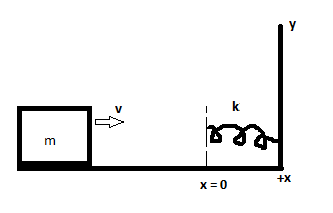# In the figure, a block of mass m = 2.5 kg slides head on into a spring of spring constant k= 320...

## Question:

In the figure, a block of mass m = 2.5 kg slides head on into a spring of spring constant k= 320 N/m. When the block stops, it has compressed the spring by 7.5 cm. The co-efficient of kinetic friction between block and floor is 0.25. While the block is in contact with the spring and being brought to rest, what is:

A) the work done by the spring force on the block?

B) the increase in thermal energy of the block-floor system?

C) the block's speed just as it reaches the spring?## Spring Force:

The spring force is the measure of the product of spring constant and the extension or compression of spring due to applied force. It is represented in terms of Newton and also named as restoring force.

Become a Study.com member to unlock this answer! Create your account

Given data

• The mass of block is {eq}m = 2.5\;{\rm{kg}} {/eq}.
• The spring constant is {eq}k = 320\;{\rm{N/m}} {/eq}.
• The length of compression is...Hooke's Law & the Spring Constant: Definition & Equation

from

Chapter 4 / Lesson 19
202K

After watching this video, you will be able to explain what Hooke's Law is and use the equation for Hooke's Law to solve problems. A short quiz will follow.